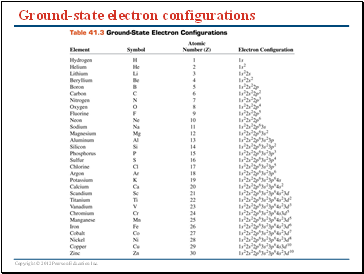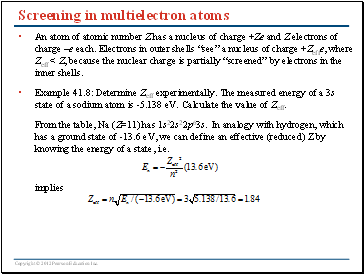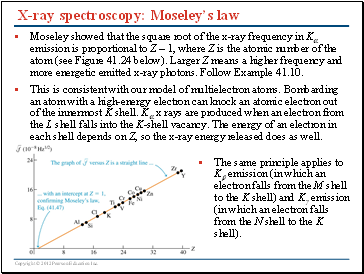# Atomic StructurePage 5

#### WATCH ALL SLIDES

Figure 41.21 (at right) is a sketch of a lithium atom, which has 3 electrons. The allowed electron states are naturally arranged in shells of different size centered on the nucleus. The n = 1 states make up the K shell, the n = 2 states make up the L shell, and so on.

Due to the Pauli exclusion principle, the 1s subshell of the K shell (n = 1, l = 0, ml = 0) can accommodate only two electrons (one with ms = + 1/2, one with ms = –1/2). Hence the third electron goes into the 2s subshell of the L shell (n = 2, l = 0, ml = 0).

Slide 25## Ground-state electron configurations

Slide 26## Screening in multielectron atoms

An atom of atomic number Z has a nucleus of charge +Ze and Z electrons of charge –e each. Electrons in outer shells “see” a nucleus of charge +Zeffe, where Zeff < Z, because the nuclear charge is partially “screened” by electrons in the inner shells.

Example 41.8: Determine Zeff experimentally. The measured energy of a 3s state of a sodium atom is -5.138 eV. Calculate the value of Zeff.

From the table, Na (Z=11) has 1s22s22p63s. In analogy with hydrogen, which has a ground state of -13.6 eV, we can define an effective (reduced) Z by knowing the energy of a state, i.e.

implies

Slide 27## X-ray spectroscopy

When atoms are bombarded with high-energy electrons, x rays are emitted. There is a continuous spectrum of x rays (described in Chapter 38) as well as strong characteristic x-ray emission at certain definite wavelengths (see the peaks labeled K and K in Figure 41.23 at right).

Atoms of different elements emit characteristic x rays at different frequencies and wavelengths. Hence the characteristic x-ray spectrum of a sample can be used to determine the atomic composition of the sample.

Slide 28X-ray spectroscopy: Moseley’s law

Moseley showed that the square root of the x-ray frequency in K emission is proportional to Z – 1, where Z is the atomic number of the atom (see Figure 41.24 below). Larger Z means a higher frequency and more energetic emitted x-ray photons. Follow Example 41.10.

This is consistent with our model of multielectron atoms. Bombarding an atom with a high-energy electron can knock an atomic electron out of the innermost K shell. K x rays are produced when an electron from the L shell falls into the K-shell vacancy. The energy of an electron in each shell depends on Z, so the x-ray energy released does as well.

The same principle applies to K emission (in which an electron falls from the M shell to the K shell) and K emission (in which an electron falls from the N shell to the K shell).

Go to page:
1  2  3  4  5  6